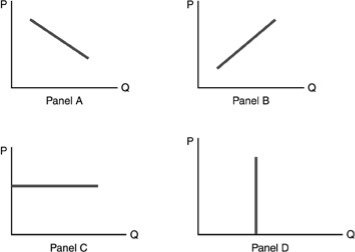/
/
/
11) Suppliers will provide more of a good when A) the
Not my Question
Flag Content

# Question : 11) Suppliers will provide more of a good when A) the

11) Suppliers will provide more of a good when

A) the market price increases.

B) the good is a normal good.

C) resource prices increase.

D) there is a decrease in demand.

12) A given supply curve illustrates

A) the relationship between price and quantity supplied.

B) the effect of a change in resource costs on quantity supplied.

C) the effect of a change in technology on quantity supplied.

D) the relationship between expected future prices and quantity supplied.

13) The data points on a supply curve come from

A) survey analysis.

B) the same place from which we get the data points on a demand curve.

C) the supply schedule.

D) companies' annual reports.

14) Other things being equal, an increase in the price of a good leads to an increase in the amount produced. This is known as

A) the law of demand.

B) the law of supply.

C) ceteris paribus.

D) equilibrium.

15) The direct relationship between changes in price and changes in quantity supplied is

A) a change in supply.

B) shown by a shift in the supply curve.

C) the law of supply.

D) the law of relative production.

16) If the price of oil rises, producers of oil will

A) increase the quantity of oil supplied.

B) supply less oil.

C) leave the amount of oil supplied unchanged.

D) cut the price.

17) The law of supply states that there is

A) an inverse relationship between price and technology, ceteris paribus.

B) a direct relationship between profit and quantity supplied, ceteris paribus.

C) no relationship between price of resources and number of suppliers, ceteris paribus.

D) a direct relationship between price and quantity supplied, ceteris paribus.

18) Regarding the law of supply, which of the following statements is correct?

A) As the price of a good or service rises, the quantity supplied will increase.

B) As the price of a good or service rises, the quantity supplied will decrease.

C) The ceteris paribus assumption does not apply.

D) As demand falls, supply rises.19) Refer to the above figure. Which panel demonstrates the law of supply?

A) Panel A

B) Panel B

C) Panel C

D) Panel D

20) The relationship between quantity supplied and the price of output is such that

A) an increase in quantity will automatically lead to a reduction in price.

B) an increase in price will lead to an increase in quantity supplied.

C) an increase in price will produce an inward shift in the supply curve.

D) quantity will decrease as the number of firms increases.

## Solution 5 (1 Ratings )

Solved
Economics 8 Months Ago 81 Views